# 帝豪EC7-RV•外观
•中控
•座椅
•细节
 本地参考价： 6.88 万元起 厂商指导价：6.88-10.18 万
 车市行情　｜　帝豪EC7-RV 降万
 最新年款： 2013款 级　别：紧凑型车 结　构：两厢 车身颜色： 红色 油　耗：6.8L/100km 排　量：1.5|1.8 内饰配色： 红黑 保　修：3年或6万公里 变速箱：MT|CVT

#### 精品国货大两厢 试驾帝豪EC718-RV#### 两款好开不贵 搭载CVT的自主品牌车#### 试驾吉利帝豪EC7-RV 全能绝非纸上谈兵1.5L 自然吸气 109马力 国IV 官方指导价 本地参考价 车型信息 2013款 1.5L 手动进取型 5挡手动 前置前驱 6.88万暂无 参数　图片　＋对比 2013款 1.5L 手动精英型 5挡手动 前置前驱 7.68万暂无 参数　图片　＋对比 2013款 1.5L 手动尊贵型 5挡手动 前置前驱 8.68万暂无 参数　图片　＋对比 2013款 1.5L 手动进取型 5挡手动 前置前驱 6.88万暂无 参数　图片　＋对比 2013款 1.5L 手动精英型 5挡手动 前置前驱 7.68万暂无 参数　图片　＋对比 2013款 1.5L 手动尊贵型 5挡手动 前置前驱 8.68万暂无 参数　图片　＋对比
 1.8L 自然吸气 139马力 国IV 官方指导价 本地参考价 车型信息 2013款 1.8L CVT精英型 CVT无级变速 前置前驱 8.98万暂无 参数　图片　＋对比 2013款 1.8L CVT尊贵型 CVT无级变速 前置前驱 10.18万暂无 参数　图片　＋对比 2013款 1.8L CVT精英型 CVT无级变速 前置前驱 8.98万暂无 参数　图片　＋对比 2013款 1.8L CVT尊贵型 CVT无级变速 前置前驱 10.18万暂无 参数　图片　＋对比

### 绕车实拍 外观中控座椅细节全部### 帝豪EC7-RV 新闻资讯#### 精品国货大两厢 试驾帝豪EC718-RV#### 两款好开不贵 搭载CVT的自主品牌车#### 试驾吉利帝豪EC7-RV 全能绝非纸上谈兵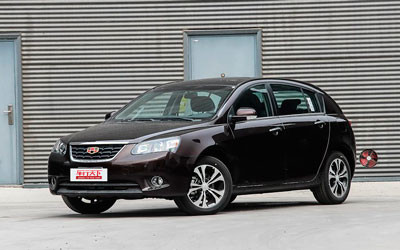#### 帝豪EC7-RV最高降3000元 交1000抵2万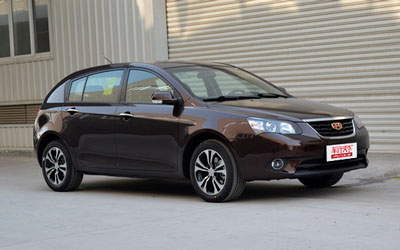#### 有望北京车展亮相 曝吉利帝豪EC7RV谍照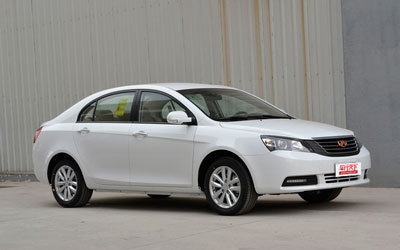#### 改款帝豪EC7 RV曝光 增加1.3T动力#### 河南华驰三大活动缤纷开幕

7月26日，河南华驰正式展开吉利新帝豪向上马拉松挑战“惠”，吉利汽车杯万元奖励寻找全城最强“地主王”活动正式鸣枪开打，吉利汽车河南华驰年...#### 给车涂点“防晒霜” 爱车夏季封釉防晒#### 吉利帝豪EC7最高优惠12000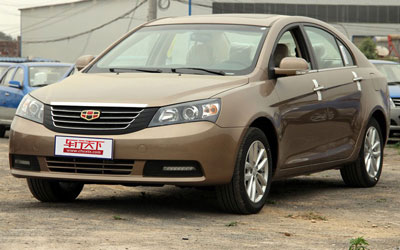#### 河南华星帝豪车型优惠万元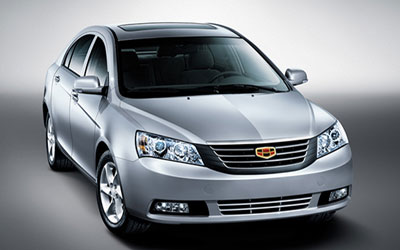#### 云阳海店帝豪EC7优惠10000#### 比一比 算一算 帝豪超值58800元### 猜你喜欢

﻿
• 快速找车
• 选择品牌
• 选择品牌
• A  奥迪
• A  阿斯顿·马丁
• A  阿尔法·罗密欧
• B  宝沃
• B  布加迪
• B  巴博斯
• B  保时捷
• B  宾利
• B  奔驰
• B  宝马
• B  本田
• B  别克
• B  标致
• B  比亚迪
• B  宝骏
• B  北汽制造
• B  北汽新能源
• B  北汽幻速
• B  北汽威旺
• B  北京汽车
• B  奔腾
• B  北汽绅宝
• B  北汽昌河
• C  长安欧尚
• C  长安
• C  长安凯程
• C  长城
• D  大众
• D  道奇
• D  DS
• D  东南
• D  东风风神
• D  东风风行
• D  东风小康
• D  东风风度
• D  东风
• F  福特
• F  丰田
• F  菲亚特
• F  法拉利
• F  福田
• F  福迪
• F  福汽启腾
• G  观致
• G  广汽传祺
• G  广汽吉奥
• G  GMC
• H  红旗
• H  汉腾汽车
• H  哈弗
• H  哈飞
• H  海格
• H  海马
• H  华颂
• H  黄海
• H  华泰
• H  恒天
• J  捷途
• J  几何汽车
• J  捷达
• J  吉利汽车
• J  捷豹
• J  Jeep
• J  江淮
• J  江铃
• J  金杯
• J  九龙
• J  金旅
• K  凯翼
• K  凯迪拉克
• K  克莱斯勒
• K  科尼塞克
• K  卡威
• K  开瑞
• L  路虎
• L  林肯
• L  劳斯莱斯
• L  兰博基尼
• L  雷克萨斯
• L  铃木
• L  领克
• L  雷诺
• L  理念
• L  力帆
• L  莲花汽车
• L  猎豹
• L  路特斯
• L  陆风
• M  马自达
• M  MG
• M  MINI
• M  玛莎拉蒂
• M  摩根
• M  迈凯轮
• N  纳智捷
• O  欧拉
• O  欧宝
• O  讴歌
• O  欧朗
• Q  奇瑞
• Q  起亚
• Q  启辰
• R  日产
• R  荣威
• R  瑞麒
• S  SERES赛力斯
• S  三菱
• S  斯威汽车
• S  萨博
• S  smart
• S  斯柯达
• S  斯巴鲁
• S  思铭
• S  双龙
• S  上汽大通
• S  双环
• T  特斯拉
• T  腾势
• W  蔚来
• W  沃尔沃
• W  WEY
• W  五菱汽车
• W  五十铃
• W  威兹曼
• W  威麟
• X  现代
• X  雪佛兰
• X  星途
• X  雪铁龙
• X  小鹏汽车
• X  西雅特
• Y  一汽
• Y  英菲尼迪
• Y  英致
• Y  依维柯
• Y  野马汽车
• Y  永源
• Z  众泰
• Z  中华
• Z  中兴
• Z  知豆
• 选择车系
• 选择车系
• 车型对比
• 选择品牌
• 选择品牌
• A  奥迪
• A  阿斯顿·马丁
• A  阿尔法·罗密欧
• B  宝沃
• B  布加迪
• B  巴博斯
• B  保时捷
• B  宾利
• B  奔驰
• B  宝马
• B  本田
• B  别克
• B  标致
• B  比亚迪
• B  宝骏
• B  北汽制造
• B  北汽新能源
• B  北汽幻速
• B  北汽威旺
• B  北京汽车
• B  奔腾
• B  北汽绅宝
• B  北汽昌河
• C  长安欧尚
• C  长安
• C  长安凯程
• C  长城
• D  大众
• D  道奇
• D  DS
• D  东南
• D  东风风神
• D  东风风行
• D  东风小康
• D  东风风度
• D  东风
• F  福特
• F  丰田
• F  菲亚特
• F  法拉利
• F  福田
• F  福迪
• F  福汽启腾
• G  观致
• G  广汽传祺
• G  广汽吉奥
• G  GMC
• H  红旗
• H  汉腾汽车
• H  哈弗
• H  哈飞
• H  海格
• H  海马
• H  华颂
• H  黄海
• H  华泰
• H  恒天
• J  捷途
• J  几何汽车
• J  捷达
• J  吉利汽车
• J  捷豹
• J  Jeep
• J  江淮
• J  江铃
• J  金杯
• J  九龙
• J  金旅
• K  凯翼
• K  凯迪拉克
• K  克莱斯勒
• K  科尼塞克
• K  卡威
• K  开瑞
• L  路虎
• L  林肯
• L  劳斯莱斯
• L  兰博基尼
• L  雷克萨斯
• L  铃木
• L  领克
• L  雷诺
• L  理念
• L  力帆
• L  莲花汽车
• L  猎豹
• L  路特斯
• L  陆风
• M  马自达
• M  MG
• M  MINI
• M  玛莎拉蒂
• M  摩根
• M  迈凯轮
• N  纳智捷
• O  欧拉
• O  欧宝
• O  讴歌
• O  欧朗
• Q  奇瑞
• Q  起亚
• Q  启辰
• R  日产
• R  荣威
• R  瑞麒
• S  SERES赛力斯
• S  三菱
• S  斯威汽车
• S  萨博
• S  smart
• S  斯柯达
• S  斯巴鲁
• S  思铭
• S  双龙
• S  上汽大通
• S  双环
• T  特斯拉
• T  腾势
• W  蔚来
• W  沃尔沃
• W  WEY
• W  五菱汽车
• W  五十铃
• W  威兹曼
• W  威麟
• X  现代
• X  雪佛兰
• X  星途
• X  雪铁龙
• X  小鹏汽车
• X  西雅特
• Y  一汽
• Y  英菲尼迪
• Y  英致
• Y  依维柯
• Y  野马汽车
• Y  永源
• Z  众泰
• Z  中华
• Z  中兴
• Z  知豆
• 选择车系
• 选择车系
• 选择车型
• 选择车型
• 意见反馈